##Desargues involution theorem related to triangles

Consider the family of all conics passing through three points {A,B,C} in general position and tangent at A to a line (t). For every line (e) not passing through any of these points, the conics of the family intersect the line at points W1, W2 in involution.
The involution f is uniquely determined by the two pairs of points (X1,X2) and (Z1,Z2) where the tangent and line BC intersect (e) and where AB, AC intersect (e) respectively.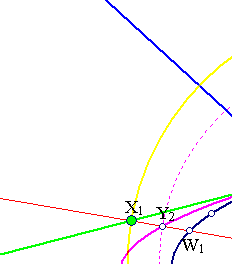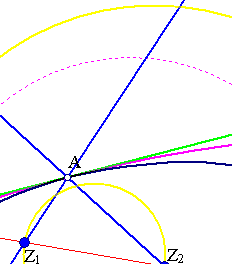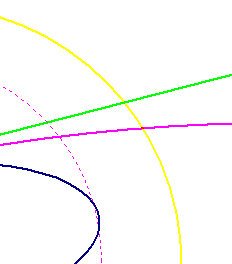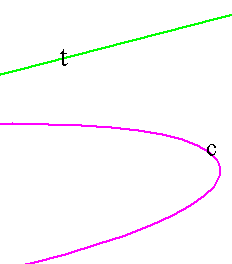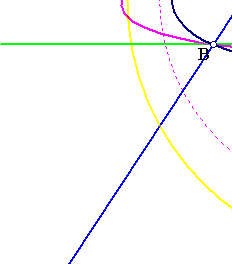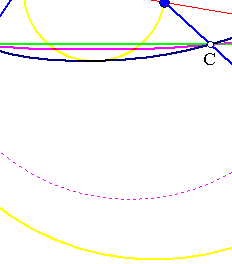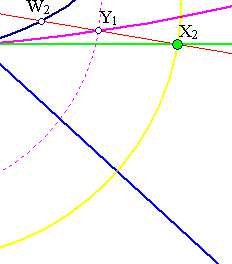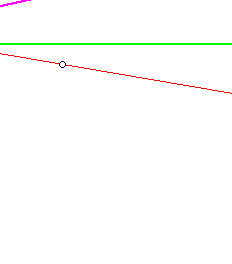This property is obtained as a special case of Desargues Involution theorem (see DesarguesInvolution.html ), in which we identify two points out of the four {A,B,C,D} involved in the original theorem. In the above figure this is done for A and D.

In the above figure I construct the variable conic (c) tangent to (t) at A and passing through B and C, using the bundle of circles generated by the two circles on diameters X1X2 and Z1Z2. To determine a conic like (c) take an arbitrary point Y1 on line (e) and the member circle of the bundle through Y1. The other intersection point Y2 of this circle with line (e) is the image of Y1 under the involution f on line (e). The five points {A,B,C,Y1,Y2} determine the conic (c). The argument with the circle-bundle is explained in InvolutionBasic2.html .
A further special case of this case of Desargues Involution theorem is obtained by letting B coincide with C. This is discussed in DesarguesInvolution3.html .

### See Also

CrossRatio0.html
DesarguesInvolution.html
DesarguesInvolution3.html
FourPtsAndTangent.html
FourPtsAndTangent2.html
HomographicRelation.html
HomographicRelationExample.html
Involution.html
InvolutionBasic.html

### References

Doerrie, Heinrich 100 Great Problems of Elementary Mathematics New York, Dover, 1965, p. 269.

 Produced with EucliDraw© http://users.math.uoc.gr/~pamfilos/